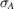International Tables for Crystallography (2006). Vol. F, ch. 15.2, pp. 325-331   | 1 | 2 | https://doi.org/10.1107/97809553602060000688

Contents

• 15.2. Model phases: probabilities, bias and maps  (pp. 325-331)
• 15.2.1. Introduction  (p. 325) | html | pdf |
• 15.2.2. Model bias: importance of phase  (p. 325) | html | pdf |
• 15.2.2.1. Parseval's theorem  (p. 325) | html | pdf |
• 15.2.3. Structure-factor probability relationships  (pp. 325-327) | html | pdf |
• 15.2.3.1. Wilson and Sim structure-factor distributions in P 1  (pp. 325-326) | html | pdf |
• 15.2.3.2. Probability distributions for variable coordinate errors  (p. 326) | html | pdf |
• 15.2.3.3. General treatment of the structure-factor distribution  (pp. 326-327) | html | pdf |
• 15.2.3.4. Estimating(p. 327) | html | pdf |
• 15.2.4. Figure-of-merit weighting for model phases  (p. 327) | html | pdf |
• 15.2.5. Map coefficients to reduce model bias  (pp. 327-328) | html | pdf |
• 15.2.5.1. Model bias in figure-of-merit weighted maps  (pp. 327-328) | html | pdf |
• 15.2.5.2. Model bias in combined phase maps  (p. 328) | html | pdf |
• 15.2.6. Estimation of overall coordinate error  (p. 328) | html | pdf |
• 15.2.7. Difference-map coefficients  (p. 328) | html | pdf |
• 15.2.8. Refinement bias  (pp. 328-329) | html | pdf |
• 15.2.9. Maximum-likelihood structure refinement  (p. 329) | html | pdf |
• References | html | pdf |
• Figures
• Fig. 15.2.2.1. Schematic illustration of the relative errors introduced by a random choice of phase or a random choice of amplitude  (p. 325) | html | pdf |
• Fig. 15.2.3.1. Centroid of the structure-factor contribution from a single atom  (p. 326) | html | pdf |
• Fig. 15.2.3.2. Schematic illustration of the general structure-factor distribution, relevant in the case of any set of independent random errors in the atomic model  (p. 327) | html | pdf |
• Fig. 15.2.4.1. Figure-of-merit weighted model-phased structure factor, obtained as the probability-weighted average over all possible phases  (p. 327) | html | pdf |